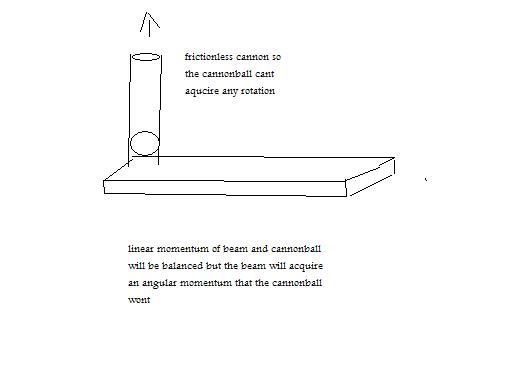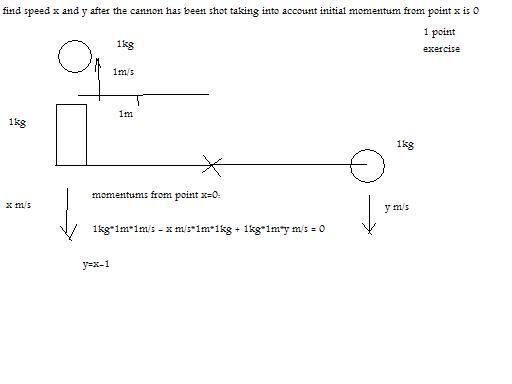# Thread: how is angular momentum conserved in this case?

1. i have an sticky platform in space and i drive over it a car

i start making a circle over the platform of 20 m and i reduce radius to 10 m, engine off

both platform and car will spin around a common axe and i will have halfed initial radius

obviously nothing speeds up

but then how is angular momentum conserved in this case?

if im not explained how angular momentum is conserved in this case i hope you respect my right to say conservation of angular momentum doesnt acomplish in certain cases

not only that, if angular momentum is not conserved in this case in my view all physics are under suspicious being all based on this premise2.

3. don't know exactly what you want here , but no gravity=no friction = no drive.4.Originally Posted by luxtpm
i have an sticky platform in space and i drive over it a car

i start making a circle over the platform of 20 m and i reduce radius to 10 m, engine off

both platform and car will spin around a common axe and i will have halfed initial radius

obviously nothing speeds up

but then how is angular momentum conserved in this case?

if im not explained how angular momentum is conserved in this case i hope you respect my right to say conservation of angular momentum doesnt acomplish in certain cases

not only that, if angular momentum is not conserved in this case in my view all physics are under suspicious being all based on this premise
Angular momentum will be conserved. All the tijme.

Your statement that "obviously nothing speeds up" is wrong.

The platform and the car will counter-rotate around a common axis during the entire exercise. At "engine off" there will be no rotation.

This scnario is an idealization of something that you can do approximately (there is some friction and a fixed axis) on a kid's playground merry-go-roud.5. do you know excalextrix?imagine a round track with half circle 1 m radius and the other half 2 m radius floating in space

you give initial energy to the car and then stop giving energy

the car when making the half circle of 2 m radius goes unpropelled at 1 m/s

so should i believe since its the most checked and verified "principle" that when the car goes to 1 m radius it will go to 2 m/s, without having spent any energy?

as i see it as the car goes trough the varying radius track floating in space, the car obviously at a constant speed unless you believe in creation of energy, will be doubling and halving angular momentum constantly in an isolated system as it doubles and halves radius of the track

it couldnt be simpler:

all right i spin a weight around an axe and when i half radius it doubled speed, i accept that

then i have a bycicle spinning around an axe, so when i half its radius of turn it will be at double speed without having spent any energy6. At "engine off" there will be no rotation.
so there was initial some rotation an amount of angular momentum and then it disappears?

then angular momentum doesnt conserve in an isolated system

if you can make an isolated system go from angular momentum 1 to ang mom 0, it doesnt matter how much energy you spent or stopped spending, ang momentum wouldnt be conserved, which would make this principle false

teoretically total angular momentum of the whole universe is 0

of course they cant find all the antimatter there should be so this is true

then maybe its not true7.Originally Posted by luxtpm
At "engine off" there will be no rotation.
so there was initial some rotation an amount of angular momentum and then it disappears?

then angular momentum doesnt conserve in an isolated system

if you can make an isolated system go from angular momentum 1 to ang mom 0, it doesnt matter how much energy you spent or stopped spending, ang momentum wouldnt be conserved, which would make this principle false

teoretically total angular momentum of the whole universe is 0

of course they cant find all the antimatter there should be so this is true

then maybe its not true
If the car/platform system starts with 0 angular momentum, then it has 0 angular momentum all the time.8.Originally Posted by luxtpm
At "engine off" there will be no rotation.
so there was initial some rotation an amount of angular momentum and then it disappears?

then angular momentum doesnt conserve in an isolated system

if you can make an isolated system go from angular momentum 1 to ang mom 0, it doesnt matter how much energy you spent or stopped spending, ang momentum wouldnt be conserved, which would make this principle false

teoretically total angular momentum of the whole universe is 0

of course they cant find all the antimatter there should be so this is true

then maybe its not true
In an isolated system, especially using the car situation you use, there are 2 or more sets of rotation that 'cancel' each other out in terms of angular momentum, in order to conserve the said angular momentum.9. i fail to see how angular momentum is conserved in this case

the car goes north, the track then goes south

the car goes north east south west

the track goes south west north east

both go clock wise

as i see it the conservation of linear momentum implies the non conservation of angular momentum in this case

its like a bycycle that is making a circle and reduces radius it wont increase speed as conservation of angular momentum would expect10. I'm not sure what you are looking for, but I think this is a good example and shows that what I think you are trying to say is wrong.

Watch figure skaters some time. When they go into a spin, then pull their legs and arms in, their rate of rotation increases dramatically.11.Originally Posted by luxtpm

as i see it the conservation of linear momentum implies the non conservation of angular momentum in this case
Conservation of linear momentum actually implies comservation of angular momentum.

Linear momentum is just mv, and angular momentum is m vXr, where X is the vector cross product and r is the vector from the point with respect to which you measure anglular momentum to the location of the mass. Since r is constant, if mv is conserved so is m vXr.12.Originally Posted by luxtpm
i fail to see how angular momentum is conserved in this case

the car goes north, the track then goes south

the car goes north east south west

the track goes south west north east

both go clock wise

as i see it the conservation of linear momentum implies the non conservation of angular momentum in this case

its like a bycycle that is making a circle and reduces radius it wont increase speed as conservation of angular momentum would expect
The problem here is you're not looking at the track from a fixed point. You're measuring the car's motion from a fixed point, but the track's from the car's point of view.

If you look at it from a fixed point of view: assume the car starts on the north end, heading east. Watch the point that starts under the car. The car goes east, south, west, north. That point that was initially under the car moves west, south, east, north. One goes clockwise, one goes counterclockwise.13. ive thought of this mental experiment which in my opinion shows a case in which angular momentum is not conserved:

im floating in space with a 70kg strip of wood under my feet

but i dont jump over the cog of the strip but over one of its extremes, in such a way as to give it a rotation

so i end up at 1 m/s towards the north balanced by the wood strip that goes at 1 m/s towards the south

but the rotation the wood strip acquired is not balanced with anything

so with an initial 0 momentum the isolated system ended up with a certain rotation or angular momentum which is not balanced, angualr momentum has been created in an isolated system14.Originally Posted by luxtpm
ive thought of this mental experiment which in my opinion shows a case in which angular momentum is not conserved:

im floating in space with a 70kg strip of wood under my feet

but i dont jump over the cog of the strip but over one of its extremes, in such a way as to give it a rotation

so i end up at 1 m/s towards the north balanced by the wood strip that goes at 1 m/s towards the south

but the rotation the wood strip acquired is not balanced with anything

so with an initial 0 momentum the isolated system ended up with a certain rotation or angular momentum which is not balanced, angualr momentum has been created in an isolated system
You can run all the thought experiments that you want to run, but it won't make any difference.

Conservation of both linear and angular momentum is a relatively simple consequence of Newton's three laws of motion.

See any book classical mechanics, Goldstein's book will do nicely.15. well isnt it called a principle?

therefore an axiom, something you SUPPOSE to start building from it

i doubt it can be demonstrated being a principle, just observed

here the concept:16.Originally Posted by luxtpm
well isnt it called a principle?

therefore an axiom, something you SUPPOSE to start building from it

i doubt it can be demonstrated being a principle, just observed

here the concept:No, it is not an axiom.

It is a theorem that is provable from Newton's three laws of motion.17.Originally Posted by luxtpm
here the concept:Draw a line between the point of rotation of the plank and the cannonball. The distance between the point of rotation and the ball is R. The rate at which this line sweep out an arc, measured in radians/sec is w. The cannon ball is mass M.

If you take the instantaneous value of R and the instantaneous value of w for the cannon ball at any point of its flight, and plug them into the Equation:You will find that the angular momentum of the cannon ball with respect to the poit of rotation of the plank will always be of equal magnitude but opposite in sign to that of the rotating plank.

Angular momentum is conserved.18. im begining to see, could someone tell me how to complete then this problem plz?19. hey plz guys could someone tell me how to solve this problem, how do i find speeds x and y?

has me anxious20.Originally Posted by luxtpm
hey plz guys could someone tell me how to solve this problem, how do i find speeds x and y?

has me anxiousit is my impression that homework problems are not to be solved on this site.21. oh its not homework its something i wonder myself to learn ,plz helpBookmarks
 Posting Permissions
 You may not post new threads You may not post replies You may not post attachments You may not edit your posts   BB code is On Smilies are On [IMG] code is On [VIDEO] code is On HTML code is Off Trackbacks are Off Pingbacks are Off Refbacks are On Terms of Use Agreement